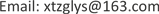﻿ 宇宙空间暴胀机制探讨 Probe into the Inflationary Mechanism of Cosmos Space

Astronomy and Astrophysics
Vol. 07  No. 03 ( 2019 ), Article ID: 31329 , 6 pages
10.12677/AAS.2019.73007

Probe into the Inflationary Mechanism of Cosmos Space

Yushan Li

Xingtai Lide Machinery Roll Manufacturing Co., Ltd., Xingtai HebeiReceived: June 26th, 2019; accepted: July 11th, 2019; published: July 18th, 2019ABSTRACT

This thesis details inferred “Planck size”, “the entropy of Blank-hole” and “the radiation temperature of Blank-hole” formula; studied the question of radiation of Blank-hole and probed into the inflationary mechanism of cosmos space.

Keywords:Cosmos Big-Bang, the Inflationary of Cosmos Space, Planck Size, Planck Black-Hole, the Entropy of Blank-Hole, the Radiation of Blank-Hole1. 文章中用到的常数

2. 普朗克尺度、普朗克黑洞

${L}_{P}=2G{m}_{P}/{c}^{2}$ (1)

mp——普朗克黑洞的质量。

$\Delta r\Delta p=h/2\text{π}$

${L}_{P}{m}_{P}c=h/2\text{π}$ (2)

(1) × (2)得： ${L}_{P}^{2}=2Gh/2\text{π}{c}^{3}=Gh/\text{π}{c}^{3}$

${L}_{P}={\left(Gh/\text{π}{c}^{3}\right)}^{1/2}$

3. 黑洞的熵及黑洞的辐射温度与辐射

3.1. 黑洞熵公式的推导

$S=nA$ (n为比例常数)

${S}_{P}=k\mathrm{log}{w}_{P}=n{A}_{P}$ (3)

(SP、wP、AP分别为普朗克黑洞的熵、微观状态数和表面积)。

(3)中对数的底数取wP时， $\mathrm{log}{w}_{P}=1$ , $k=n{A}_{P}$ , $n=k/{A}_{P}$

$S=nA=Ak{c}^{3}/4Gh$ (4)

$S=4\text{π}kG{m}^{2}/ch$ (m为黑洞的质量) (5)

( $S={k}_{B}\mathrm{ln}w$ )玻尔兹曼常数kB之关系。

$k={m}_{P}{c}^{2}/{T}_{P}$ (6)

$PV=\left(NR/{N}_{0}\right)T$

$PV=N{k}_{B}T$ (7)

${P}_{P}{V}_{P}=N{k}_{B}{T}_{P}$

${P}_{P}{V}_{P}={k}_{B}{T}_{P}$

$Q={m}_{P}{c}^{2}+{P}_{P}{V}_{P}$

${m}_{P}{c}^{2}-{P}_{P}{V}_{P}=0$${P}_{P}{V}_{P}={m}_{P}{c}^{2}$

3.2. 黑洞的辐射温度

${T}_{B}=\text{d}Q/\text{d}S=\text{d}\left(m{c}^{2}\right)/\text{d}S={c}^{2}\text{d}m/\text{d}S$

${T}_{B}={c}^{2}/\left(\text{d}S/\text{d}m\right)$

${T}_{B}={c}^{2}/\left(8\text{π}kGm/ch\right)$

${T}_{B}={c}^{3}h/8\text{π}kGm$ (9)

${T}_{P}={m}_{P}{c}^{2}/{k}_{B}$

3.3. 黑洞的辐射(霍金辐射)

4. 宇宙空间暴胀机制

${V}^{r-1}T=衡量$

${T}_{S}={\left({L}_{P}/{R}_{P}\right)}^{2}{T}_{P}$

${T}_{B}=\left(Gh/2\text{π}{c}^{3}{L}_{P}{R}_{B}\right){T}_{P}$

${R}_{S}=ab{L}_{P}$${R}_{B}=b{L}_{P}$ (a、b为系数，取正数)于是根据(10)、(11)有：

${T}_{S}={\left(1/ab\right)}^{2}{T}_{P}$ , ${T}_{B}=\left(1/2b\right){T}_{P}$

2) 当 $b=2$ 时， $a=1$${R}_{S}={R}_{B}=2{L}_{P}$ 空间半径与黑洞半径相等；

3) 当 $b>2$ 时， $a<1$${R}_{S}<{R}_{B}$ 空间半径小于黑洞半径。

1) 表示空间与黑洞等温同步膨胀，空间半径始终大于黑洞半径；

2) 表示空间与黑洞同步膨胀至半径均等于二倍的普朗克尺度，此时的温度为： ${T}_{B}={T}_{P}/4$

3) 表示如果继续膨胀，黑洞的半径将比空间的半径要大，温度也要比空间的温度高。然而这种情况是不可能发生的，此刻黑洞将会以霍金辐射的方式剧烈蒸发，从而致使空间以爆发式增长，即发生暴胀。

${R}_{S}={R}_{B}=a{L}_{P}$ (a为系数，取正数) 于是根据(10)、(11)分别有：

${T}_{S}={\left({L}_{P}/{R}_{S}\right)}^{2}{T}_{P}={T}_{P}/{a}^{2}$ (12)

${T}_{B}=\left({L}_{P}/2{R}_{B}\right){T}_{P}={T}_{P}/2a$ (13)

(12)/(13)得： ${T}_{S}/{T}_{B}=2/a$

2) 当 $a=2$${R}_{S}={R}_{B}=2{L}_{P}$ 时， ${T}_{S}={T}_{B}$ ，空间温度与黑洞温度相等；

3) 当 $a>2$${R}_{S}={R}_{B}>2{L}_{P}$ 时， ${T}_{S}<{T}_{B}$ ，空间温度低于黑洞温度。

(1)、(2)两种情况说明，空间与黑洞等径膨胀直至 ${R}_{S}={R}_{B}=2{L}_{P}$

(3)表明，如果继续膨胀，空间温度将低于黑洞温度，此刻黑洞将会以霍金辐射的方式剧烈蒸发，从而使空间的爆发式增长，以至发生暴胀。

5. 结论

1) 普朗克尺度 ${L}_{P}={\left(Gh/\text{π}{c}^{3}\right)}^{1/2}$

2) 黑洞的熵 $S=Ak{c}^{3}/4Gh=4\text{π}kG{m}^{2}/ch$ ，其中的玻尔兹曼常数k就是自然对数条件下( $S={k}_{B}\mathrm{ln}w$ )的玻尔兹曼常数kB；黑洞的辐射温度 ${T}_{B}={c}^{3}h/8\text{π}kGm$

3) 普朗克黑洞的熵等于自然对数条件下的玻尔兹曼常数，即 ${S}_{P}={k}_{B}$ ；普朗克黑洞的微观状态数等于自然数，即 ${w}_{P}=\text{e}$

4) 黑洞可通过空间的量子涨落而产生辐射。

5) 宇宙原始空间与黑洞的相互作用使空间发生暴胀。

Probe into the Inflationary Mechanism of Cosmos Space[J]. 天文与天体物理, 2019, 07(03): 65-70. https://doi.org/10.12677/AAS.2019.73007

1. 1. 程守洙, 江之永, 主编. 普通物理学[M]. 北京: 人民教育出版社, 1978